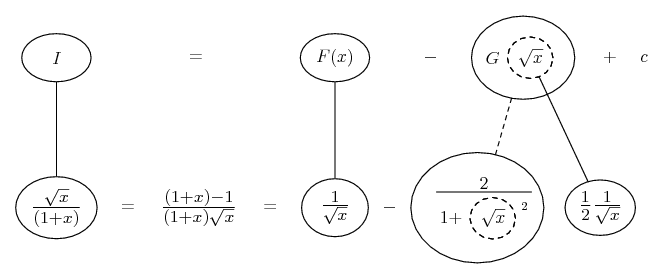# Thread: Integration Question help please

1. ## Integration Question help please

----------------------------------------------------------
Integrate x^(1/2) / (1 + x) dx
-----------------------------------
I substituted x^(1/2) = sin y

so it becomes Integral of 2*tany*siny dy

then integration by parts its

2*(tany*cosy - Integral of (-secy) dy)

= 2ln| sec y + tan y | - 2 siny

then substituting x back in

= 2ln| (1 + x^(1/2)) / (1 - x)^(1/2) | - 2x^(1/2)

but the answer in the back of the book is

2x^(1/2) - 2tan^-1( x^(1/2) )

If anyone can tell me what I'm doing wrong it will be much appreciated

2. Divide top and bottom (of the integrand) by root x, then re-write the top (now x) as 1 + x -1. Will add a pic...

Edit:

Just in case a picture helps (same as redsox below, I guess)...... where... is the chain rule. Straight continuous lines differentiate downwards (integrate up) with respect to x, and the straight dashed line similarly but with respect to the dashed balloon expression (which is the inner function of the composite, subject to the chain rule).

__________________________________________

Don't integrate - balloontegrate!

http://www.ballooncalculus.org/forum/top.php

Draw balloons with LaTeX: http://www.ballooncalculus.org/asy/doc.html

3. you wan to let sqrt(x) = tan(y)

I think you mistakenly used 1 + sin^2(y) = cos^2(y) in the denominator

but 1+ sin^2(y) = 2 - cos^2(y)

See attachment

4. the next to last line in attachment should read

sqrt(x)dx/(1+x) = 2sec^2(y) -2

5.Originally Posted by kinetix2006----------------------------------------------------------
Integrate x^(1/2) / (1 + x) dx
-----------------------------------
I substituted x^(1/2) = sin y

so it becomes Integral of 2*tany*siny dy

then integration by parts its

2*(tany*cosy - Integral of (-secy) dy)

= 2ln| sec y + tan y | - 2 siny

then substituting x back in

= 2ln| (1 + x^(1/2)) / (1 - x)^(1/2) | - 2x^(1/2)

but the answer in the back of the book is

2x^(1/2) - 2tan^-1( x^(1/2) )

If anyone can tell me what I'm doing wrong it will be much appreciated
$\displaystyle \int\frac{\sqrt{x}}{1+x}\,dx$

I would do it like this (to avoid using trig terms, which can get messy):

Let $\displaystyle x=u^2$. Thus $\displaystyle dx=2u\,du$.

Now you have $\displaystyle \int\frac{2u^2}{1+u^2}\,du = \int\frac{2u^2+2-2}{1+u^2}\,du =$$\displaystyle \int\frac{2u^2+2}{u^2+1}-\frac{2}{1+u^2}\,du = \int 2-2\frac{1}{1+u^2}\,du = 2u-2\arctan(u)$

Now sub back in $\displaystyle u=\sqrt{x}$ and add $\displaystyle C$ and you're done.
$\displaystyle \boxed{\int\frac{\sqrt{x}}{1+x}\,dx = 2\sqrt{x}-2\arctan(\sqrt{x})+C}$

,

,

### Integral tan y / cos y

Click on a term to search for related topics.

#### Search Tags

integration, question